﻿ [转]10种机器学习算法Part.1-FunnyWeb

# [转]10种机器学习算法Part.1

2016-10-17 00:54:21955 views

http://www.analyticsvidhya.com/blog/2015/08/common-machine-learning-algorithms/

1. 线性回归

2. 逻辑回归

3. 决策树

4. SVM

5. 朴素贝叶斯

6. K最近邻算法

7. K均值算法

8. 随机森林算法

9. 降维算法

## 1、线性回归 Linear Regression

• Y：因变量

• a：斜率

• x：自变量

• b ：截距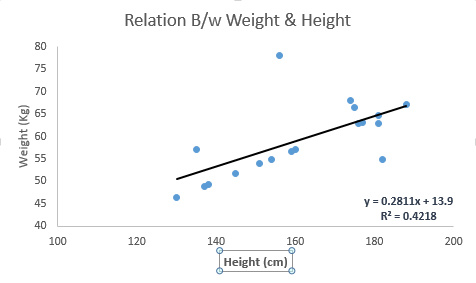Python Code

```#Import Library
#Import other necessary libraries like pandas, numpy...
from sklearn import linear_model
#Identify feature and response variable(s) and values must be numeric and numpy arrays
x_train=input_variables_values_training_datasets
y_train=target_variables_values_training_datasets
x_test=input_variables_values_test_datasets
# Create linear regression object
linear = linear_model.LinearRegression()
# Train the model using the training sets and check score
linear.fit(x_train, y_train)
linear.score(x_train, y_train)
#Equation coefficient and Intercept
print('Coefficient:', linear.coef_)
print('Intercept:', linear.intercept_)
#Predict Output
predicted= linear.predict(x_test)```

R Code

```#Load Train and Test datasets
#Identify feature and response variable(s) and values must be numeric and numpy arrays
x_train <- input_variables_values_training_datasets
y_train <- target_variables_values_training_datasets
x_test <- input_variables_values_test_datasets
x <- cbind(x_train,y_train)# Train the model using the training sets and check scorelinear <- lm(y_train ~ ., data = x)
summary(linear)#Predict Output
predicted= predict(linear,x_test)```

## 2、逻辑回归 Logistic Regression

odds= p/ (1-p) = probability of event occurrence / probability of not event occurrence

ln(odds) = ln(p/(1-p))

logit(p) = ln(p/(1-p)) = b0+b1X1+b2X2+b3X3....+bkXk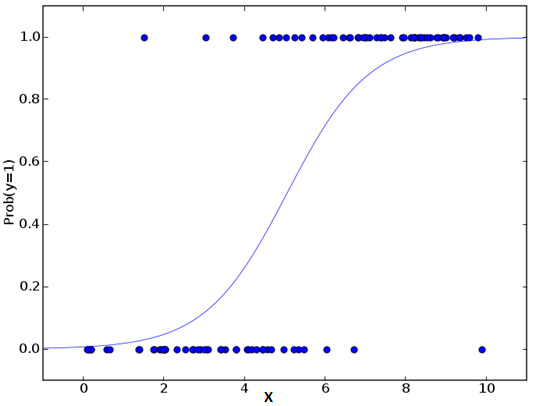Python Code

```#Import Library
from sklearn.linear_model
import LogisticRegression
#Assumed you have, X (predictor) and Y (target) for training data set and x_test(predictor) of test_dataset
# Create logistic regression object
model = LogisticRegression()
# Train the model using the training sets and check score
model.fit(X, y)
model.score(X, y)
#Equation coefficient and Interceptprint('Coefficient:
', model.coef_)
print('Intercept:
', model.intercept_)
#Predict Output
predicted= model.predict(x_test)```

R Code

```x <- cbind(x_train,y_train)# Train the model using the training sets and check scorelogistic <- glm(y_train ~ ., data = x,family='binomial')
summary(logistic)#Predict Output
predicted= predict(logistic,x_test)```

• 加入交互项

• 精简模型特性

• 使用正则化方法

• 使用非线性模型

## 3、决策树 Decision Tree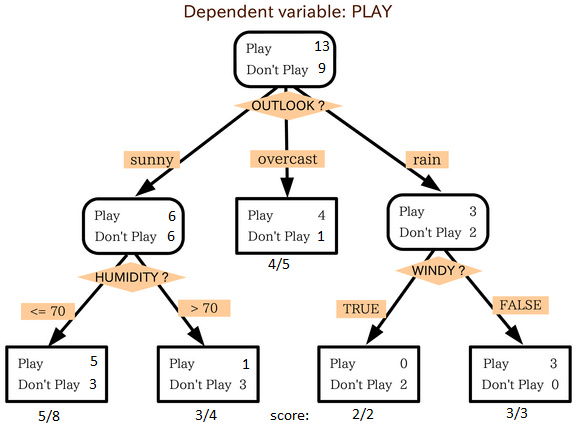#### Python Code

```#Import Library
#Import other necessary libraries like pandas, numpy...
from sklearn import tree
#Assumed you have, X (predictor) and Y (target) for training data set and x_test(predictor) of test_dataset# Create tree object
model = tree.DecisionTreeClassifier(criterion='gini')
# for classification, here you can change the algorithm as gini or entropy (information gain) by default it is gini
# model = tree.DecisionTreeRegressor() for regression
# Train the model using the training sets and check score
model.fit(X, y)
model.score(X, y)
#Predict Output
predicted= model.predict(x_test)```

R Code

```library(rpart)
x <- cbind(x_train,y_train)# grow tree
fit <- rpart(y_train ~ ., data = x,method="class")summary(fit)#Predict Output
predicted= predict(fit,x_test)```

## 4、支持向量机 SVM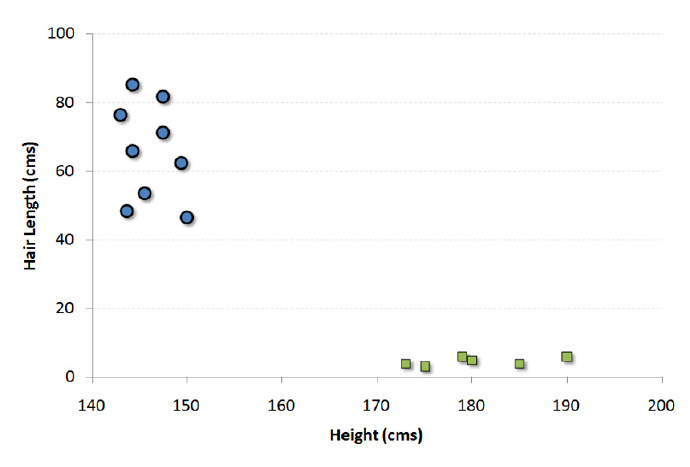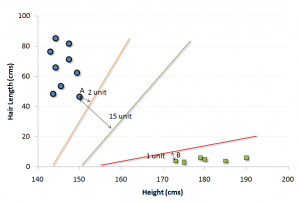上面示例中的黑线将数据分类优化成两个小组，两组中距离最近的点（图中A、B点）到达黑线的距离满足最优条件。这条直线就是我们的分割线。接下来，测试数据落到直线的哪一边，我们就将它分到哪一类去。

• 比起之前只能在水平方向或者竖直方向画直线，现在你可以在任意角度画线或平面。

• 游戏的目的变成把不同颜色的球分割在不同的空间里。

• 球的位置不会改变。

#### Python Code

```#Import Library
from sklearn import svm
#Assumed you have, X (predictor) and Y (target) for training data set and x_test(predictor) of test_dataset
# Create SVM classification object
model = svm.svc()
# there is various option associated with it, this is simple for classification. You can refer link, for more detail.
# Train the model using the training sets and check score
model.fit(X, y)
model.score(X, y)
#Predict Output
predicted= model.predict(x_test)```

R Code

```library(e1071)
x <- cbind(x_train,y_train)# Fitting model
fit <-svm(y_train ~ ., data = x)summary(fit)#Predict Output
predicted= predict(fit,x_test)```

## 5、朴素贝叶斯 Naive Bayes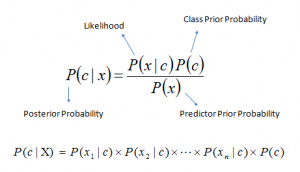• P(c|x) 是已知预示变量（属性）的前提下，类（目标）的后验概率

• P(c) 是类的先验概率

• P(x|c) 是可能性，即已知类的前提下，预示变量的概率

• P(x) 是预示变量的先验概率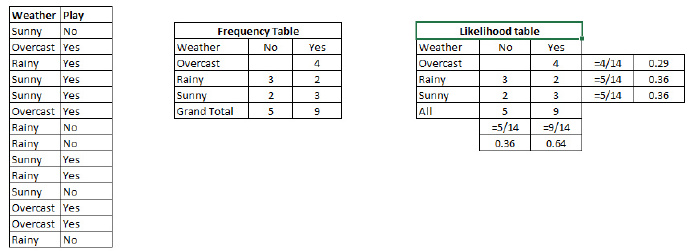#### Python Code

```#Import Library
from sklearn.naive_bayes
import GaussianNB
#Assumed you have, X (predictor) and Y (target) for training data set and x_test(predictor) of test_dataset
# Create SVM classification object
model = GaussianNB()
# there is other distribution for multinomial classes like Bernoulli Naive Bayes, Refer link
# Train the model using the training sets and check score
model.fit(X, y)
#Predict Output
predicted= model.predict(x_test)```

R Code

```library(e1071)
x <- cbind(x_train,y_train)# Fitting model
fit <-naiveBayes(y_train ~ ., data = x)summary(fit)#Predict Output
predicted= predict(fit,x_test)```

﻿